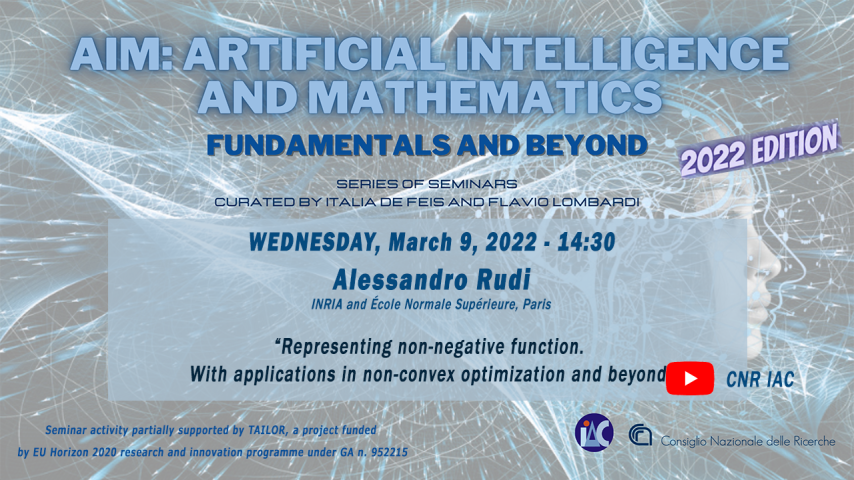# Alessandro Rudi - Representing non-negative function. With applications in non-convex optimization and beyondMany problems in applied mathematics are expressed naturally in terms of non-negative functions. While linear models are well suited to represent functions with output in R, being at the same time very expressive and flexible, the situation is different for the case of non-negative functions where the existing models lack one of good properties.In this talk we present a rather flexible and expressive model for non-negative functions. We will show direct applications in probability representation and non-convex optimization. In particular, the model allows to derive an algorithm for non-convex optimization that is adaptive to the degree of differentiability of the objective function and achieves optimal rates of convergence. Finally, we show how to apply the same technique to other interesting problems in applied mathematics that can be easily expressed in terms of inequalities.

Data inizio